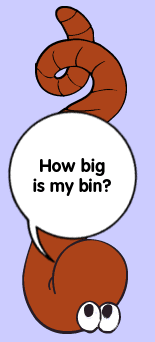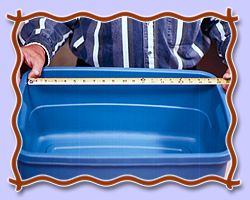Now that you've got my "room" made, you need to fill it with the stuff I love and need. The first thing I need is bedding. To figure out how much I need, you need to know the volume of my bin in cubic feet. This is how you're going to measure the cubic feet of bin space. First, measure the depth, width and length of my bin in inches. Then multiply the depth by width by length to get the cubic inches in my bin. For example, let's say the bin is 8 inches deep, 24 inches wide and 24 inches long. That's 4,608 cubic inches. You got this answer by multiplying depth (8 inches) by width (24 inches) by length (24 inches). Now we need to convert it to find out how many cubic feet are in my box. A cubic foot is 1 foot deep by 1 foot wide by 1 foot long. There are 12 inches in a foot. So take 12 inches x 12 inches x 12 inches to get 1,728 cubic inches in a cubic foot. So how many cubic feet are in a bin that is 4,608 cubic inches? Divide the cubic inches in the bin by the cubic inches in a cubic foot to get the answer. 4,608 ÷ 1,728 = 2.67 cubic feet Let's try a few more problems . . .